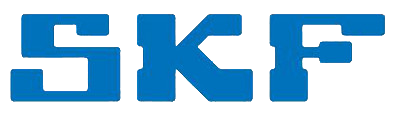# In Line Skate BearingsManufacturer Product Image Item Name Price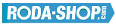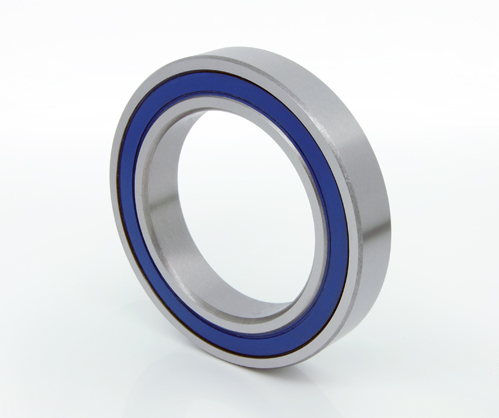### 608-2RS

Miniature Metric Series Chrome Steel Rubber Seals on Both sides Dimension d=8.00 Dimension D=22.00 Dimension B=7.00 Dinamic Load Rating=3293 Static Load Rating=1379 Weight=0.014gr. Speed limit Grease=34.0 Speed limit Oil=40.0
inside diameter = 8.00
outside diameter = 22.00
width = 7.00
€2.24### 608-2Z

Miniature Metric Series Chrome Steel Metallic Shields on both sides Dimension d=8.00 Dimension D=22.00 Dimension B=7.00 Dinamic Load Rating=3293 Static Load Rating=1379 Weight=0.014gr. Speed limit Grease=34.0 Speed limit Oil=40.0
inside diameter = 8.00
outside diameter = 22.00
width = 7.00
€2.24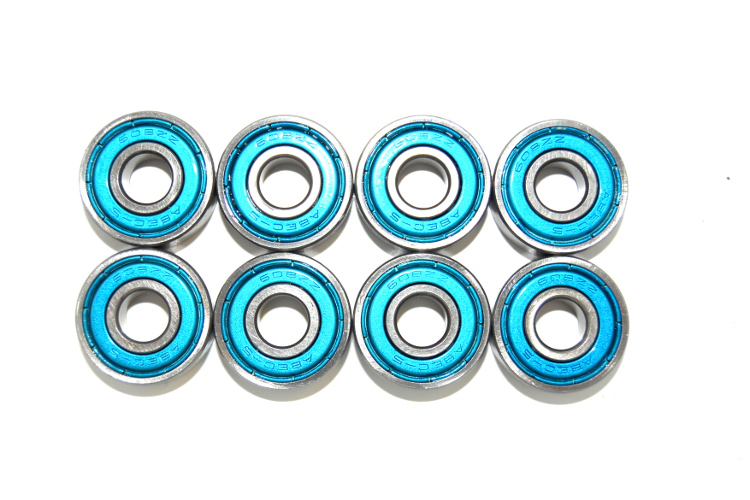### 608-2Z-ABEC5-BLUE (PACK 8)

8-PIECES PACK Miniature Metric Series Chrome Steel ABEC-5 Metallic Shields on both sides Dimension d=8.00 Dimension D=22.00 Dimension B=7.00 Dinamic Load Rating=3293 Static Load Rating=1379 Weight/Unit=0.014Kgs Speed limit Grease=34.0 Speed limit Oil=40.0
inside diameter = 8
outside diameter = 22
width = 7
€14.00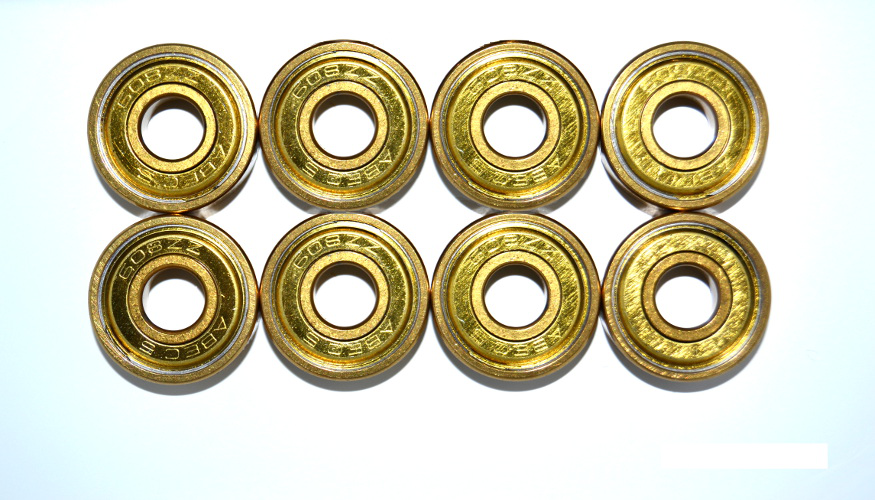### 608-2Z-ABEC5-GOLD(PACK 8)

8-PIECES PACK Miniature Metric Series Chrome Steel ABEC-5 Metallic Shields on both sides Dimension d=8.00 Dimension D=22.00 Dimension B=7.00 Dinamic Load Rating=3293 Static Load Rating=1379 Weight=0.014Kgs Speed limit Grease=34.0 Speed limit Oil=40.0
inside diameter = 8
outside diameter = 22
width = 7
€14.00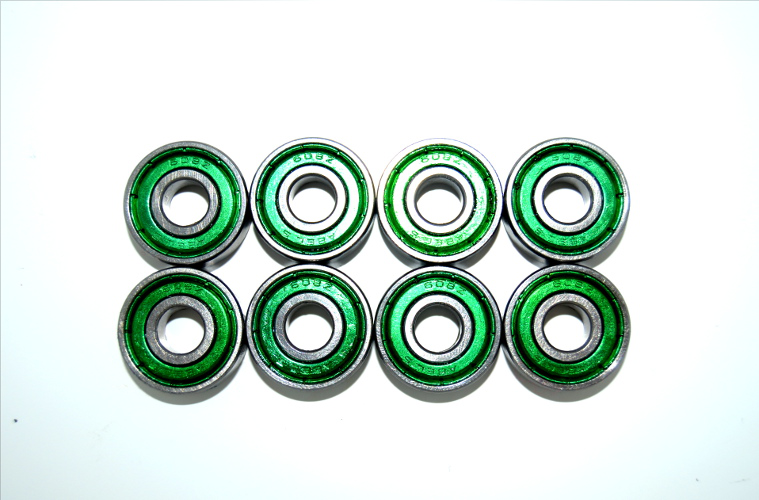### 608-2Z-ABEC5-GREEN(PACK 8)

8-PIECES PACK Miniature Metric Series Chrome Steel ABEC-5 Metallic Shields on both sides Dimension d=8.00 Dimension D=22.00 Dimension B=7.00 Dinamic Load Rating=3293 Static Load Rating=1379 Weight=0.014Kgs Speed limit Grease=34.0 Speed limit Oil=40.0
inside diameter = 8
outside diameter = 22
width = 7
€14.00### 608-2Z-ABEC5-LIGHT GREEN(PACK 8)

8-PIECES PACK Miniature Metric Series Chrome Steel ABEC-3 Metallic Shields on both sides Dimension d=8.00 Dimension D=22.00 Dimension B=7.00 Dinamic Load Rating=3293 Static Load Rating=1379 Weight=0.014Kgs Speed limit Grease=34.0 Speed limit Oil=40.0
inside diameter = 8
outside diameter = 22
width = 7
€14.00### 608-2Z-ABEC7-RED(PACK 8)

8-PIECES PACK Miniature Metric Series Chrome Steel ABEC-7 Metallic Shields on both sides Dimension d=8.00 Dimension D=22.00 Dimension B=7.00 Dinamic Load Rating=3293 Static Load Rating=1379 Weight=0.014Kgs Speed limit Grease=34.0 Speed limit Oil=40.0
inside diameter = 8
outside diameter = 22
width = 7
€16.00### 608-2ZW6

Miniature Metric Series Chrome Steel Metallic Shields on both sides Dimension d=8.00 Dimension D=22.00 Dimension B=6.00 Dinamic Load Rating=3293 Static Load Rating=1379 Weight=0.013gr. Speed limit Grease=34.0 Speed limit Oil=40.0
inside diameter = 8.00
outside diameter = 22.00
width = 6.00
€2.24